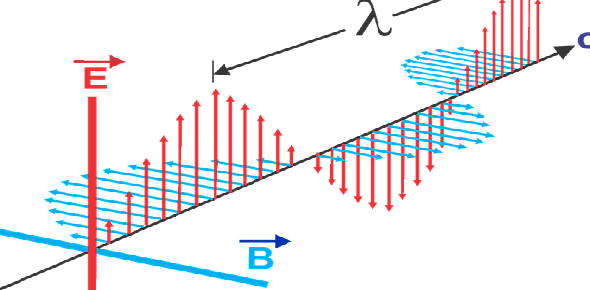Why do electrical currents display magnetic properties? - ProProfs DiscussTopicsMoreProductsMore+ Ask Question

# Why do electrical currents display magnetic properties?Change Image    Delete

Asked by I. Klose, Last updated: Feb 09, 2020

###Request 0FollowShareAnswer AnonymouslyAnswer LaterCopy Link2#### J.Spencer

Knowledge Enthusiast, Knows A Lot of Stuff.

J.Spencer, Knowledge enthusiast, Tokyo

The answer is actually quite simple. It is the fundamental property of a charge to generate a magnetic field. That’s all there is to it. Yes, there is a relatively tough scientific explanation to it. For that, you would need a good knowledge of calculus especially partial differentials, know what Lorentz force is and also the concept of special relativity.

Simply speaking Lorentz force is the sum of the force exerted by Electric fields and Magnetic fields at a given point having, let’s say q charge.

So, the Lorentz force at a given point is equal to,

F= qE + qv B

Where E is the electric field, B is the magnetic field and v is the velocity of the particle(since there is no magnetic force on a stationary particle).

Taking this equation in the space-time dimension, where charge q is at the position at (xp, yp, zp) and the electric field and magnetic field on it are equal to E(tp, xp, yp, zp) and B(tp, xp, yp, zp)

The net Lorentz force on the particle is,

F= q (E (tp, xp, yp, zp) + vB (tp, xp, yp, zp) )

This is where special relativity comes in. Since that is a lengthy explanation with way too many derivations to explain, lets simply explain it in words. For example, in the dimensions of both space and time, an electric field acting on a particle in space has a different field acting on it if observed from another point in space. Basically, your inertial point of reference changes the nature of the force that’s acting on your particle.

I’ll give another example. Consider two points R1 and R2. Both points are different points of observation for the same particle. The electric field seen from R1 on the particle might seem like the magnetic field seen from R2 particle. In essence what this means is that both electric field and magnetic field are one and the same force. It's just the where you observe it from that matters. Change your observation point and your field changes.

Applying this concept to your above equation, you get,

E2 = ^(E1 + v B1) - (^-1) (E1.v/ ||v||)v

One more thing to remember all fields, velocities in the equation are variable and ^ is a frequency. I hope that answered your question.2#### J. Harty

Have keen interest in writing, traveller by heart.

J. Harty, Writer, M.A, Chula VistaMagnetism is a category of physical phenomena that are mediated by magnetic fields. Electric currents and the magnetic movements of elementary particles give rise to a magnetic field, which acts on other currents magnetic movements.

Anti-ferromagnetic materials such as chromium and spin glasses have a more complex relationship with magnetic fields. The pressure of a magnet on paramagnetic, diamagnetic, and antiferromagnetic substances is usually too weak to be detected and can only be diagnosed by laboratory instruments. In everyday life, these substances are described as non-magnetic.

The magnetic action of a material depends on its structure, particularly it is electron configuration. At elevated temperatures, random thermal motion makes it more difficult for electrons to maintain alignment.Search for Google imagesSelect a recommended image
Upload from your computerCancelSearch for Google imagesSelect a recommended image
Upload from your computerCancelSearch for Google imagesSelect a recommended image
Upload from your computerCancel